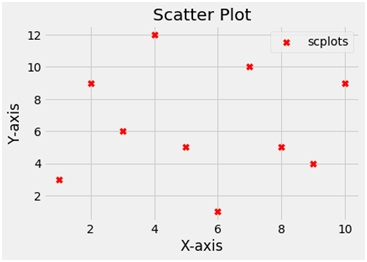# Python | Create a scatter plot using matplotlib.pyplot

Here, we are implementing a python program to create a scatter plot using matplotlib.pyplot.
Submitted by Ayush Sharma, on November 19, 2018

Problem statement: Write a program in python (using matplotlib.pyplot) to create a scatter plot.

Use of scatter plot: Scatter plots are usually used to compare two variables (three if you are plotting in 3 dimensions), looking for correlation or groups.

Program:

```import matplotlib.pyplot as plt

x = [1,2,3,4,5,6,7,8,9,10]
y = [3,9,6,12,5,1,10,5,4,9]

plt.scatter(x,y, label='scplots', color='r', s=60, marker="X")

plt.xlabel('X-axis')
plt.ylabel('Y-axis')
plt.title('Scatter Plot')
plt.legend()
plt.show()
```

OutputExplanation:

Python library matplotlib.pyplot is used to draw the above chart. Two random variables x and y are taken with random values. The scatter function plots a scatter plot. The scatter function takes 2 arguments i.e. x and y and a label variable gives the label to the plot. To name the axes X-axis and Y-axis functions are used and to give the title to the plot the title function is used. To show the legend the legend function is used and finally to show the plot the show function.

Preparation